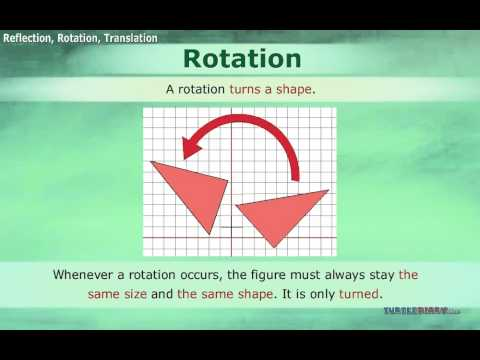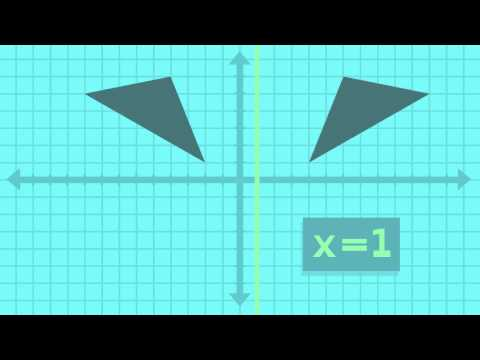## Reflection of Geometrical Figures

Subject: Compulsory Maths

#### Overview

The reflection of a geometrical figure means the formation of the image of the figure after reflecting the figure about the line of reflection.

#### Reflection in Geometrical Figures

The reflection of a geometrical figure means the formation of the image of the figure after reflecting the figure about the line of reflection.

Properties of Reflection

1. There should be equal distance between the geometrical figure and its image from the line of reflection.
2. The areas of the geometrical figure and its image are equal.
3. The appearance of the image of a figure is opposite to figure.

Reflection of geometrical figures using coordinates

Here, we can find the co-ordinates of the images of geometrical figures formed due to the reflection by x-axis and y-axis separately. In this case, x-axis and y-axis are called the axis of reflection.

1. X-axis as the axis of reflection
When a geometrical figure is reflected about x-axis as the axis of reflection, the x-coordinate of the image remains the same and the sign of y-coordinate of the image as changed.

2. Y-axis as the axis of reflection
When a geometrical figure is reflected about y-axis as the axis of reflection, the sign of x-coordinate of the image is changed but the y-coordinate of the image remains the same.

##### Things to remember
• Properties of Reflection

1. There should be equal distance between the geometrical figure and its image from the line of reflection.
2. The areas of the geometrical figure and its image are equal.
3. The appearance of the image of a figure is opposite to figure.
• It includes every relationship which established among the people.
• There can be more than one community in a society. Community smaller than society.
• It is a network of social relationships which cannot see or touched.
• common interests and common objectives are not necessary for society.
##### Videos for Reflection of Geometrical Figures##### Translations Reflections and Rotations##### Math Shorts Episode 4 - Reflection

Solution:

A(2,-2)$\rightarrow$A’(-2,-2)

B(5,3)$\rightarrow$B’(-5,3)

C(1,4)$\rightarrow$C’(-1,4)

So, the coordinates of the vertices of image $\triangle$A’B’C’ are A(-2,-2), B(-5,3) and C(-1,4).

Solution:

A(-2,3)$\rightarrow$A’(-2,-2)

B(2,1)$\rightarrow$B’(2,-1)

C(4,4)$\rightarrow$C’(4,-4)

So, the coordinates of the vertices of image $\triangle$A’B’C’ are A(-2,-3), B(2,-1) and C(4,-4).

When the coordinates of the images of geometrical figures formed due to the reflection by x - axis and y - axis, then in this case x-axis and y-axis are called the axis of reflection.

Solution: